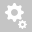##Recently viewed tickets# [BOUNDARY]     Reference for soil theory (Integral Bridge Spring Support)

Function Location: Boundary > Spring Supports >Integral Bridge.

midas Civil has a function called the Integral Bridge Spring Support. An integral bridge is one in which the bridge deck and its supporting abutments and piers are integrated without expansion joints to absorb the deformation of the bridge deck using the flexibility of the abutments and piers. The prime concern in integral bridges is the effects of temperature variations on the deformation of bridge deck. Expansion and contraction of the bridge deck affects the backfill soil adjacent to the abutments. Backfill compaction due to a deck expansion and soil slide due to a deck contraction is repeated. Due to the repeated backfill compaction and soil slide, the modulus of subgrade reaction and the pressure distribution of backfill vary with depth.

A Cycle is the period from a deck expansion to a deck contraction. If cycles are repeated infinitely, the modulus of subgrade reaction of backfill becomes constant. Using the formulation proposed by B.M. Lehane, soil springs can be assigned.

To account for this characteristic of the soil, lateral springs are modeled as compression-only springs and vertical springs are modeled as linear elastic springs.

midas Civil or midas Gen defines the nonlinear spring along with the pile, when we use the pile spring function.

Software will generate the vertical and horizontal spring automatically when you use this function.First of all, the vertical spring is a linear elastic one which doesn’t consider the nonlinearity of soil material. We, in general, do not take the nonlinearity into account, because this is about the settlement of pile within the elastic range in terms of behavior.
The equation which is described in the manual is as the following.Regardless of the soil type, we can simply define the stiffness based on the horizontal subgrade reaction, Kh, called k-value. I believe that the engineer must know about this how we can get this value. Basically it must come from lap, or field test like SPT(Standard penetration test).
Ko is the earth pressure coefficient which can define the relation between normal stress and horizontal stress of the soil (Horizontal = Ko x Normal). This is the definition of the Ko.
In case of D x I (Diameter X Length) is just the area (the equivalent area of pile tip supported). So we can simply estimate the vertical stiffness based on the horizontal subgrade reaction.
Again, this is just definition of Ko, nobody should ask where we can find the reference, who suggested the equation.

You can also refer to the following link, quite well summarized for the horizontal behavior of soil and subgrade reaction along with the references.
https://connect.ncdot.gov/resources/Geological/Documents/3B-1_A49_p-y-methodology.pdf

Secondly, for the horizontal spring, it represents the interaction between soil and structure considering the nonlinearity of soil material. For this , we have to perform the test, called “lateral load test”. From this test we can get the nonlinear curve like the following image. This is what is called “p-y curve”, the relative displacement against horizontal load applied. Once the soil reaches to the critical state, the soil will not be able to resist the applied load anymore. This is what the soil behaves in general, and this is why we should consider the nonlinear spring when it comes to deal with the soil-structure interaction.Some experts had performed this test with various types of soils and figured out, for instance, this curve might be able to represent this type of soil. Based on the test results, they could suggest the equations can define the soil behavior for some representative soil types such as sand and clay. Almost all about geotechnical ones come from empirical something, not from a specific theory.
Since it is not easy to test using all types of soil for all areas every time, now we are using the equations they suggested to simulate the soil behavior with FE software.
The following equations are the ones you can find in Civil Online manual for Sand and Clay respectively.If you want to look at where the above equations come from, please find the enclosed manual , Chapter 1.4 for sand and Chapter 1.6 for stiff and soft clay respectively.
You can find the reference and the equations suggested by Grandfather of geotechnical engineering like the followings.
You might feel like it looks different because the different symbols have used, but if you take a close look at each term, you will definitely figure out , those are the exactly same equations based on the same theory.

In summary,the engineer should input some soil parameters. And the software will use the parameter to define the “p-y (soil response) curve”, so can check the deformation of structures considering the nonlinearity of soil materials.If you use the geotechnical software, you do not need to think about the above equations, since you can simply assign one of the soil material models like Mohr coulomb, soft clay..etc according to the soil types you have.
Creation date: 9/4/2016 10:20 AM ()      Updated: 11/18/2020 5:39 PM (Pratap)